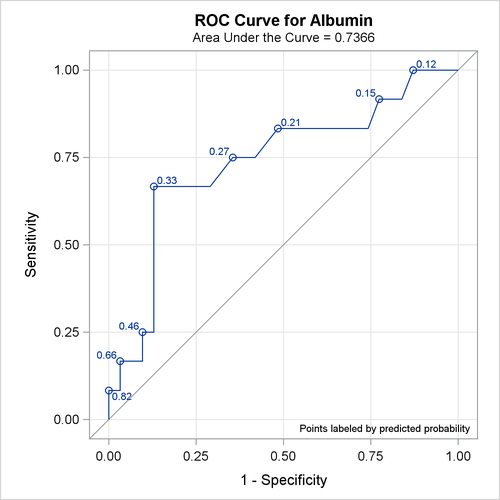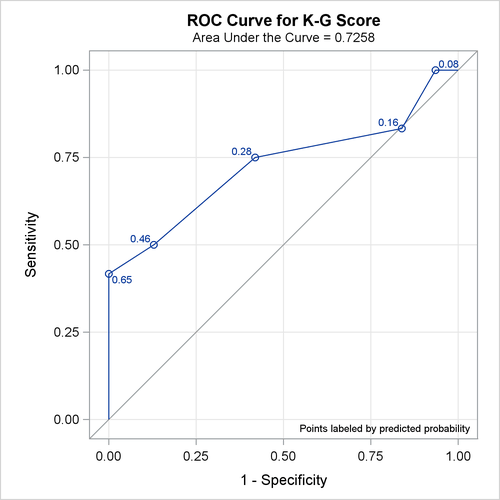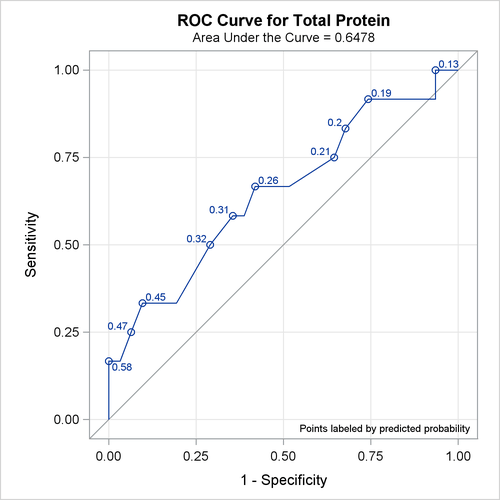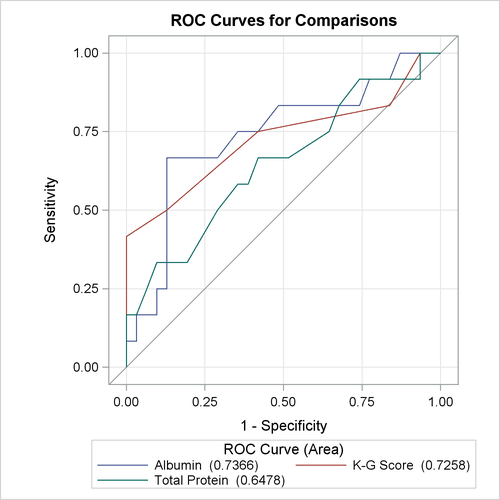Example 54.8 Comparing Receiver Operating Characteristic Curves

DeLong, DeLong, and Clarke-Pearson (1988) report on 49 patients with ovarian cancer who also suffer from an intestinal obstruction. Three (correlated) screening tests are measured to determine whether a patient will benefit from surgery. The three tests are the K-G score and two measures of nutritional status: total protein and albumin. The data are as follows:

data roc;
input alb tp totscore popind @@;
totscore = 10 - totscore;
datalines;
3.0 5.8 10 0   3.2 6.3  5 1   3.9 6.8  3 1   2.8 4.8  6 0
3.2 5.8  3 1   0.9 4.0  5 0   2.5 5.7  8 0   1.6 5.6  5 1
3.8 5.7  5 1   3.7 6.7  6 1   3.2 5.4  4 1   3.8 6.6  6 1
4.1 6.6  5 1   3.6 5.7  5 1   4.3 7.0  4 1   3.6 6.7  4 0
2.3 4.4  6 1   4.2 7.6  4 0   4.0 6.6  6 0   3.5 5.8  6 1
3.8 6.8  7 1   3.0 4.7  8 0   4.5 7.4  5 1   3.7 7.4  5 1
3.1 6.6  6 1   4.1 8.2  6 1   4.3 7.0  5 1   4.3 6.5  4 1
3.2 5.1  5 1   2.6 4.7  6 1   3.3 6.8  6 0   1.7 4.0  7 0
3.7 6.1  5 1   3.3 6.3  7 1   4.2 7.7  6 1   3.5 6.2  5 1
2.9 5.7  9 0   2.1 4.8  7 1   2.8 6.2  8 0   4.0 7.0  7 1
3.3 5.7  6 1   3.7 6.9  5 1   3.6 6.6  5 1
;

In the following statements, the NOFIT option is specified in the MODEL statement to prevent PROC LOGISTIC from fitting the model with three covariates. Each ROC statement lists one of the covariates, and PROC LOGISTIC then fits the model with that single covariate. Note that the original data set contains six more records with missing values for one of the tests, but PROC LOGISTIC ignores all records with missing values; hence there is a common sample size for each of the three models. The ROCCONTRAST statement implements the nonparameteric approach of DeLong, DeLong, and Clarke-Pearson (1988) to compare the three ROC curves, the REFERENCE option specifies that the K-G Score curve is used as the reference curve in the contrast, the E option displays the contrast coefficients, and the ESTIMATE option computes and tests each comparison. With ODS Graphics enabled, the plots=roc(id=prob) specification in the PROC LOGISTIC statement displays several plots, and the plots of individual ROC curves have certain points labeled with their predicted probabilities.

ods graphics on;
proc logistic data=roc plots=roc(id=prob);
model popind(event='0') = alb tp totscore / nofit;
roc 'Albumin' alb;
roc 'K-G Score' totscore;
roc 'Total Protein' tp;
roccontrast reference('K-G Score') / estimate e;
run;
ods graphics off;

The initial model information is displayed in Output 54.8.1.

Output 54.8.1: Initial LOGISTIC Output

The LOGISTIC Procedure

Model Information
Data Set WORK.ROC
Response Variable popind
Number of Response Levels 2
Model binary logit
Optimization Technique Fisher's scoring

 Number of Observations Read 43 43

Response Profile
Ordered
Value
popind Total
Frequency
1 0 12
2 1 31

Probability modeled is popind=0.

Score Test for Global Null
Hypothesis
Chi-Square DF Pr > ChiSq
10.7939 3 0.0129

For each ROC model, the model fitting details in Outputs Output 54.8.2, Output 54.8.4, and Output 54.8.6 can be suppressed with the ROCOPTIONS(NODETAILS) option; however, the convergence status is always displayed.

The ROC curves for the three models are displayed in Outputs Output 54.8.3, Output 54.8.5, and Output 54.8.7. Note that the labels on the ROC curve are produced by specifying the ID=PROB option, and are the predicted probabilities for the cutpoints.

Output 54.8.2: Fit Tables for Popind=Alb

Model Convergence Status
Convergence criterion (GCONV=1E-8) satisfied.

Model Fit Statistics
Criterion Intercept Only Intercept and
Covariates
AIC 52.918 49.384
SC 54.679 52.907
-2 Log L 50.918 45.384

Testing Global Null Hypothesis: BETA=0
Test Chi-Square DF Pr > ChiSq
Likelihood Ratio 5.5339 1 0.0187
Score 5.6893 1 0.0171
Wald 4.6869 1 0.0304

Analysis of Maximum Likelihood Estimates
Parameter DF Estimate Standard
Error
Wald
Chi-Square
Pr > ChiSq
Intercept 1 2.4646 1.5913 2.3988 0.1214
alb 1 -1.0520 0.4859 4.6869 0.0304

Odds Ratio Estimates
Effect Point Estimate 95% Wald
Confidence Limits
alb 0.349 0.135 0.905

Output 54.8.3: ROC Curve for Popind=AlbOutput 54.8.4: Fit Tables for Popind=Totscore

Model Convergence Status
Convergence criterion (GCONV=1E-8) satisfied.

Model Fit Statistics
Criterion Intercept Only Intercept and
Covariates
AIC 52.918 46.262
SC 54.679 49.784
-2 Log L 50.918 42.262

Testing Global Null Hypothesis: BETA=0
Test Chi-Square DF Pr > ChiSq
Likelihood Ratio 8.6567 1 0.0033
Score 8.3613 1 0.0038
Wald 6.3845 1 0.0115

Analysis of Maximum Likelihood Estimates
Parameter DF Estimate Standard
Error
Wald
Chi-Square
Pr > ChiSq
Intercept 1 2.1542 1.2477 2.9808 0.0843
totscore 1 -0.7696 0.3046 6.3845 0.0115

Odds Ratio Estimates
Effect Point Estimate 95% Wald
Confidence Limits
totscore 0.463 0.255 0.841

Output 54.8.5: ROC Curve for Popind=TotscoreOutput 54.8.6: Fit Tables for Popind=Tp

Model Convergence Status
Convergence criterion (GCONV=1E-8) satisfied.

Model Fit Statistics
Criterion Intercept Only Intercept and
Covariates
AIC 52.918 51.794
SC 54.679 55.316
-2 Log L 50.918 47.794

Testing Global Null Hypothesis: BETA=0
Test Chi-Square DF Pr > ChiSq
Likelihood Ratio 3.1244 1 0.0771
Score 3.1123 1 0.0777
Wald 2.9059 1 0.0883

Analysis of Maximum Likelihood Estimates
Parameter DF Estimate Standard
Error
Wald
Chi-Square
Pr > ChiSq
Intercept 1 2.8295 2.2065 1.6445 0.1997
tp 1 -0.6279 0.3683 2.9059 0.0883

Odds Ratio Estimates
Effect Point Estimate 95% Wald
Confidence Limits
tp 0.534 0.259 1.099

Output 54.8.7: ROC Curve for Popind=TpAll ROC curves being compared are also overlaid on the same plot, as shown in Output 54.8.8.

Output 54.8.8: Overlay of All Models Being ComparedOutput 54.8.9 displays the association statistics, and displays the area under the ROC curve along with its standard error and a confidence interval for each model in the comparison. The confidence interval for Total Protein contains 0.50; hence it is not significantly different from random guessing, which is represented by the diagonal line in the preceding ROC plots.

Output 54.8.9: ROC Association Table

ROC Association Statistics
ROC Model Mann-Whitney Somers' D
(Gini)
Gamma Tau-a
Area Standard
Error
95% Wald
Confidence Limits
Albumin 0.7366 0.0927 0.5549 0.9182 0.4731 0.4809 0.1949
K-G Score 0.7258 0.1028 0.5243 0.9273 0.4516 0.5217 0.1860
Total Protein 0.6478 0.1000 0.4518 0.8439 0.2957 0.3107 0.1218

Output 54.8.10 shows that the contrast used ’K-G Score’ as the reference level. This table is produced by specifying the E option in the ROCCONTRAST statement.

Output 54.8.10: ROC Contrast Coefficients

ROC Contrast Coefficients
ROC Model Row1 Row2
Albumin 1 0
K-G Score -1 -1
Total Protein 0 1

Output 54.8.11 shows that the 2-degrees-of-freedom test that the ’K-G Score’ is different from at least one other test is not significant at the 0.05 level.

Output 54.8.11: ROC Test Results (2 Degrees of Freedom)

ROC Contrast Test Results
Contrast DF Chi-Square Pr > ChiSq
Reference = K-G Score 2 2.5340 0.2817

Output 54.8.12 is produced by specifying the ESTIMATE option in the ROCCONTRAST statement. Each row shows that the curves are not significantly different.

Output 54.8.12: ROC Contrast Row Estimates (1-Degree-of-Freedom Tests)

ROC Contrast Estimation and Testing Results by Row
Contrast Estimate Standard
Error
95% Wald
Confidence Limits
Chi-Square Pr > ChiSq
Albumin - K-G Score 0.0108 0.0953 -0.1761 0.1976 0.0127 0.9102
Total Protein - K-G Score -0.0780 0.1046 -0.2830 0.1271 0.5554 0.4561